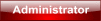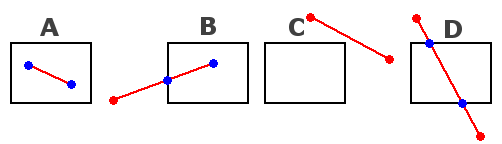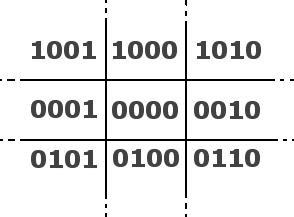TIN HỌC ỨNG DỤNG 2 - K11
Bạn hãy đăng ký làm thành viên để có thể xem các thông tin trong lớp và viết bài trong diễn đàn.

Không những thế, sau khi đăng ký bạn sẽ nhận được sự hỗ trợ của diễn đàn nhiều hơn.Introduction

When drawing a 2D line on screen, it might happen that one or both of the endpoints are outside the screen
while a part of the line should still be visible. In that case, an efficient algorithm
is needed to find two new endpoints that are on the edges on the screen,
so that the part of the line that's visible can now be drawn. This way, all those points
of the line outside the screen are clipped away and you don't need to waste
any execution time on them.

A good clipping algorithm is the Cohen-Sutherland algorithm. The function containing
this algorithm is already included in QuickCG in the file QuickCG.cpp and is called clipLine.
You pass the coordinates of the old line, and the coordinates of the new line by reference so that
the function can return the coordinates of the new line by changing those parameters.

Clipping is a very important aspect of 3D graphics, and so in the 3D Lines tutorial,
this 2D Clipping function is used often.
Cohen Sutherland
Clipping Algorithm

When drawing a 2D line, if one endpoint of the line is outside the screen, and the other
inside, you have to clip the line so that only the part of it
that's inside the screen remains. Even if both endpoints are
outside the screen, it's still possible that a part of the line
should be visible. The clipping algorithm needs to find new
endpoints of the lines, that are inside or on the edges of the
screen. Here are a few cases, where the black rectangle represents
the screen, in red are the old endpoints, and in blue the ones
after clipping:• Case A: both endpoints are inside the screen, no clipping
needed.
• Case B: one endpoint outside the screen, that one had to be
clipped
• Case C: both endpoint are outside the screen, and no part of
the line is visible, don't draw it at all.
• Case D: both endpoint are outside the screen, and a part of the
line is visible, clip both endpoints and draw it.

There are tons of different cases, each endpoint can be inside the
screen, left of it, right of it, above, below, etc... The Cohen
Sutherland Clipping Algorithm can recognize these cases quite
efficiently and do the clipping. The algorithm divides the 2D space
in 9 regions:The center region is the screen, and the other 8 regions are on
different sides outside the screen. Each region is given a binary
number, called an "outcode". The codes are chosen as follows:

• If the region is above the screen, the first bit is 1
• If the region is below the screen, the second bit is 1
• If the region is to the right of the screen, the third bit is
1
• If the region is to the left of the screen, the fourth bit is
1

Obviously an area can't be to the left and the right at the same
time, or above and below it at the same time, so the third and
fourth bit can't be 1 together, and the first and second bit can't
be 1 together. The screen itself has all 4 bits set to 0.

Both endpoints of the line can lie in any of these 9 regions, and
there are a few trivial cases:

• If both endpoints are inside or on the edges of the screen, the
line is inside the screen or clipped, and can be drawn. This case
is the trivial accept.
• If both endpoints are on the same side of the screen (e.g.,
both endpoints are above the screen), certainly no part of the line
can be visible on the screen. This case is thetrivial
reject
, and the line doesn't have to be drawn.

These two cases can easily be detected thanks to the outcodes of
the regions:

• Trivial Accept: both endpoints have to be in the region with
code 0000, so the trivial accept case only happens if code1 |
code2 == 0, where code1 and code2 are the codes of both
endpoints of the line, and '|' is the binary OR operator, which can
only return 0 if both codes are 0.
• Trivial Reject: because of the way the codes of the regions
were chosen, only if both endpoints of the line are on the same
side of the region, both codes will have two corresponding bits
that are both 1. For example, only if both endpoints are on the
left of the screen, the fourth bit of both codes is 1. So, the
trivial reject case is detected if code1 & code2 != 0,
where & is the binary AND operation. The binary AND operation
only returns a non zero result if two corresponding bits are
1.

All other cases (i.e. no trivial reject and no trivial accept) have
to be turned into a trivial case by doing one clip operation. The
Cohen Sutherland algorithm is a loop, that does only one clipping
operation at the time. It can clip one endpoint of the line, and
only clip it to a vertical or horizontal region border. In many
cases, it has to clip multiple times before it can finally detect
if the line is to be accepted, or rejected. It never has to be
clipped more than about 4 times though, so it's quite fast.

The function that uses the Cohen Sutherland Clipping Algorithm is
the clipLine function from QuickCG, which is in the QuickCG.cpp
file. It uses an auxiliary function, findRegion, that returns the
binary code of the region a given endpoint is in. For example to
set the second bit to 1, you have to 'OR' the code with 4 (the
first bit represents 8, the second 4, the third 2, and the firth
(the primary bit) represents 1).

Code:
`[table class="codetable"][tr][td style="vertical-align: top;"]int findRegion(int x, int y){    int code=0;    if(y >= h)    code |= 1; //top    else if( y < 0)    code |= 2; //bottom    if(x >= w)    code |= 4; //right    else if ( x < 0)    code |= 8; //left    return(code);}[/td][/tr][/table]`

The clipLine function loop starts with detecting if there's a
trivial case:

Code:
`[table class="codetable"][tr][td style="vertical-align: top;"]bool clipLine(int x1, int y1, int x2, int y2, int & x3, int & y3, int & x4, int & y4){    int code1, code2, codeout;    bool accept = 0, done=0;    code1 = findRegion(x1, y1); //the region outcodes for the endpoints    code2 = findRegion(x2, y2);    do  //In theory, this can never end up in an infinite loop, it'll always come in one of the trivial cases eventually    {        if(!(code1 | code2)) accept = done = 1;  //accept because both endpoints are in screen or on the border, trivial accept        else if(code1 & code2) done = 1; //the line isn't visible on screen, trivial reject[/td][/tr][/table]`

If no trivial case was detected, the line has to be clipped. Only
one of the 4 possible clipping operations is done at the time. To
clip, one coordinate of 1 endpoint is set to one of the borders of
the regions, which also happen to be the coordinates of borders of
the screen, and the other coordinate of that point is recalculated
by filling in the equation of the line. To detect which clipping
operation has to be performed, an endpoint that's not inside the screen has to be
chosen. The code of that endpoint is called codeout, and is set to
either code1 or code2 depending on which of those isn't zero.

Code:
`[table class="codetable"][tr][td style="vertical-align: top;"]        else  //if no trivial reject or accept, continue the loop        {            int x, y;            codeout = code1 ? code1 : code2;            if(codeout & 1) //top            {                x = x1 + (x2 - x1) * (h - y1) / (y2 - y1);                y = h - 1;            }            else if(codeout & 2) //bottom            {                x = x1 + (x2 - x1) * -y1 / (y2 - y1);                y = 0;            }            else if(codeout & 4) //right            {                y = y1 + (y2 - y1) * (w - x1) / (x2 - x1);                x = w - 1;            }            else //left            {                y = y1 + (y2 - y1) * -x1 / (x2 - x1);                x = 0;            }[/td][/tr][/table]`

The part above calculated the new coordinates of the clipped point,
and now the coordinates have to be set to endpoint1 or endpoint2
depending which endpoint codeout represented. This is also the end
of the loop, after this either the line entered a trivial case, or
it's still not a trivial case and the loop is performed again to do
more clipping.

Code:
`[table class="codetable"][tr][td style="vertical-align: top;"]            if(codeout == code1) //first endpoint was clipped            {                x1 = x; y1 = y;                code1 = findRegion(x1, y1);            }            else //second endpoint was clipped            {                x2 = x; y2 = y;                code2 = findRegion(x2, y2);            }        }    }    while(done == 0);[/td][/tr][/table]`

After the loop and thus the clipping is done, the function sets the
4 coordinates of the new line (which were passed to the function by
reference) and returns whether or not we had a trivial accept or a
trivial reject.

Code:
`[table class="codetable"][tr][td style="vertical-align: top;"]    if(accept)    {        x3 = x1;        x4 = x2;        y3 = y1;        y4 = y2;        return 1;    }    else    {        x3 = x4 = y3 = y4 = 0;        return 0;    }}[/td][/tr][/table]`

Ký tên:
Gemini Photo là nơi cung cấp gói dịch vụ chụp ảnh thời trang, chụp ảnh giá rẻ, chụp ảnh ngoài trời, chụp ảnh cưới,chụp ảnh bé yêu ... dành cho cộng đồng đặc biệt là giới trẻ

Add: 37/144 Ngô Gia Tự - Long Biên - Hà Nội
SĐT: 0166 623 5623
Map: tinyurl.com/map-geminiphoto
Báo giá: tinyurl.com/geminiphoto-baogia

Các bạn có thể coi thêm tại đây:
facebook.com/GeminiPhotoStudio

Bài viết liên quan

Quyền hạn của bạn:

You cannot reply to topics in this forum